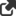# Futures SpreadsheetsThis archive contains spreadsheet files created for Futures magazine, as detailed in Gibbons Burke's monthly column, The Computerized Trader. Includes the following files:

VAMI - A way of reporting fund performance whereby each reporting period is indexed at 100 or 1000

T-TEST - a macro-based sheet to calculate trade statistics (could be useful for regular statistics t-score work)

SMA-EMA - Provides a derivation and associated charting of SPX with Simple and Exponential Moving Averages

SHARPER - calculates a Sharper ration which is supposed to improve on the Sharpe ratio not by not penalizing profitable periods (while penalizing deep extended periods of drawdown more than shallow)

RETURNS - simple sheet which calculates the total and annualized return based on starting and ending amounts and dates

RETRACE - a Fibonacci Calculator which automatically calculates the correct retracement and projection numbers along with the current level of retracement

OTPIMALF - Optimal F is a money management concept introduced by Ralph Vince. Vince says that for any given trading system trading a given market, there is some optimal fraction (f) of a trader's equity stake that should be put at risk which will produce the highest return after a series of trades. If a trader is not trading at this optimal f value, he/she is going to significantly reduce the trading results. These worksheets operate in tandem to calculate the optimal f value for a given trading track record. (Note: includes a readme file)

INDEXER - Comparing Apples to Apples

APRIL15 - Easing the sting of April 15th (Tax day trade)